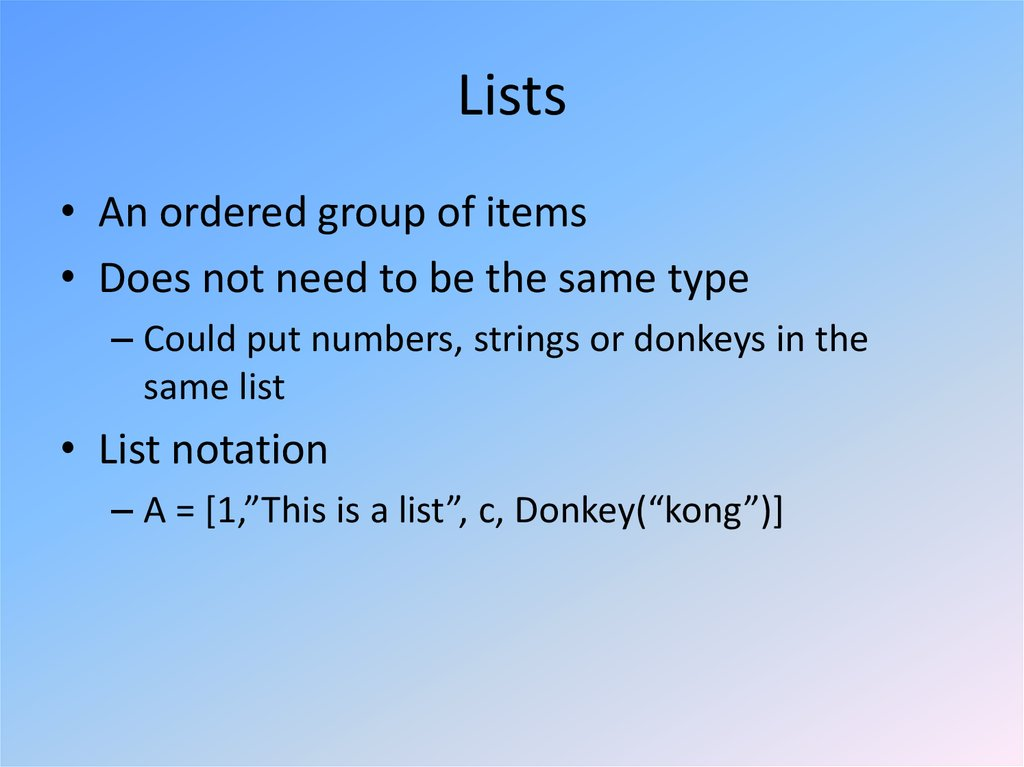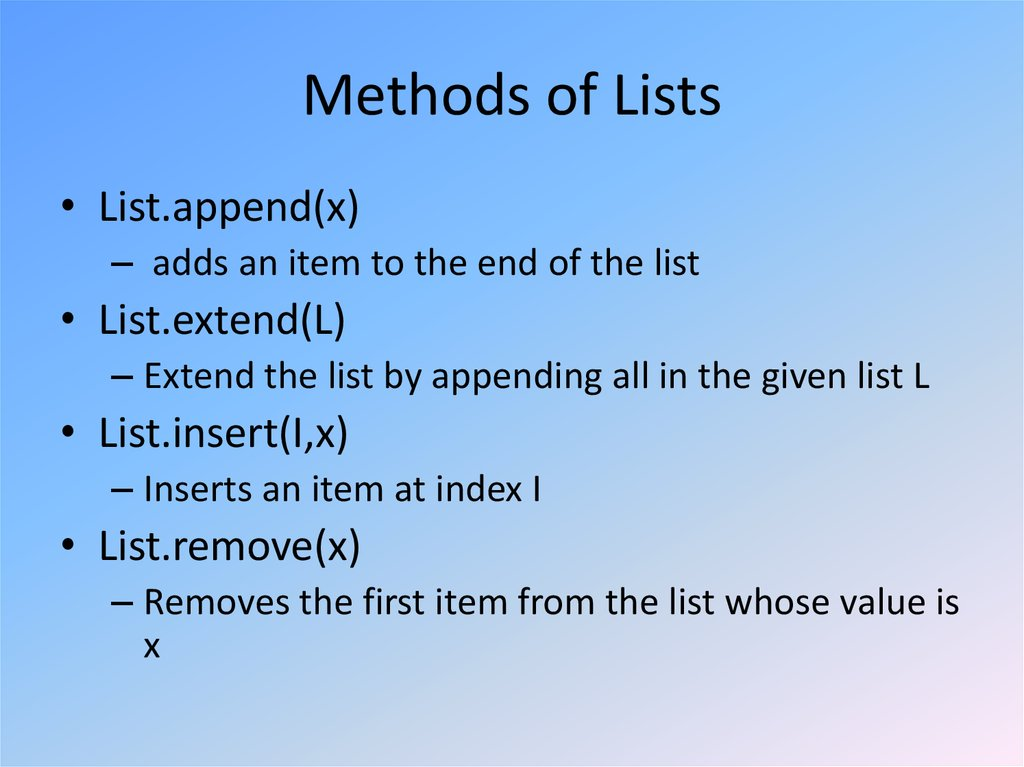# Python Data Structures

By Greg Felber

## 2. Lists

• An ordered group of items
• Does not need to be the same type
– Could put numbers, strings or donkeys in the
same list
• List notation
– A = [1,”This is a list”, c, Donkey(“kong”)]

## 3. Methods of Lists

• List.append(x)
– adds an item to the end of the list
• List.extend(L)
– Extend the list by appending all in the given list L
• List.insert(I,x)
– Inserts an item at index I
• List.remove(x)
– Removes the first item from the list whose value is
x

## 4. Examples of other methods

• a = [66.25, 333, 333, 1, 1234.5] //Defines List
– print a.count(333), a.count(66.25), a.count('x') //calls method
– 210
//output
• a.index(333)
– //Returns the first index where the given value appears
– 1 //ouput
• a.reverse()
– a
– [333, 1234.5, 1, 333, -1, 66.25]
//Reverses order of list
//Prints list a
//Ouput
• a.sort()
– a
– [-1, 1, 66.25, 333, 333, 1234.5]
//Prints list a
//Output

## 5. Using Lists as Stacks

• The last element added is the first element retrieved
• To add an item to the stack,
append() must be used
– stack = [3, 4, 5]
– stack.append(6)
– Stack is now [3, 4, 5, 6]
• To retrieve an item from the top of the stack, pop must
be used
– Stack.pop()
– 6 is output
– Stack is now [3, 4, 5] again

## 6. Using Lists as Queues

• First element added is the first element
retrieved
• To do this collections.deque
must be implemented

## 7. List Programming Tools

• Filter(function, sequence)
– Returns a sequence consisting of the items from
the sequence for which function(item) is true
– Computes primes up to 25

## 8. Map Function

• Map(function, sequence)
– Calls function(item) for each of the sequence’s
items
– Computes the cube for the range of 1 to 11

## 9. Reduce Function

• Reduce(function, sequence)
– Returns a single value constructed by calling the
binary function (function)
– Computes the sum of the numbers 1 to 10

## 10. The del statement

• A specific index or range can be deleted

## 11. Tuples

• Tuple
– A number of values separated by commas
– Immutable
• Cannot assign values to individual items of a tuple
• However tuples can contain mutable objects such as lists
– Single items must be defined using a comma
• Singleton = ‘hello’,

## 12. Sets

• An unordered collection with no duplicate
elements
• Basket = [‘apple’, ‘orange’, ‘apple’, ‘pear’]
• Fruit
– Set([‘orange’, ‘apple’, ‘pear’])

## 13. Dictionaries

• Indexed by keys
– This can be any immutable type (strings, numbers…)
– Tuples can be used if they contain only immutable objects

## 14. Looping Techniques

• Iteritems():
– for retrieving key and values through a dictionary

## 15. Looping Techniques

• Enumerate():
– for the position index and values in a sequence

## 16.

• Zip():
– for looping over two or more sequences

## 17. Comparisons

• Operators “in” and “not in” can be used to see
if an item exists in a sequence
• Comparisons can be chained
– a < b == c
• This tests whether a is less than b and that b equals c